Click in-app to access the full platform documentation for your version of DataRobot.

# Optimization metrics¶

The following table lists all metrics, with a short description, available from the Optimization Metric dropdown. The sections below the table provide more detailed explanations, leveraging information from across the internet.

Tip

Remember that the metric DataRobot chooses for scoring models is usually the best selection. Changing the metric is advanced functionality and recommended only for those who understand the metrics and the algorithms behind them. For information on how recommendations are made, see Recommended metrics.

For weighted metrics, the weights are the result of smart downsampling and/or specifying a value for the Advanced options weights parameter. The metric then takes those weights into account. Metrics used are dependent on project type, either R (regression), C (binary classification), or M (multiclass).

What are true/false negatives and true/false positives?

Consider the following definitions:

• True means the prediction was correct; false means the prediction was incorrect.
• Positive means the model predicted positive; negative means it predicted negative.

Based on those definitions:

• True positives are observations correctly predicted as positive.
• True negatives are observations correctly predicted as negative.
• False positives are observations incorrectly predicted as positive.
• False negatives are observations incorrectly predicted as negative.
Display Full name Description Project type
Accuracy Accuracy Computes subset accuracy; the set of labels predicted for a sample must exactly match the corresponding set of labels in y_true. Binary classification, multiclass
AUC/Weighted AUC Area Under the (ROC) Curve Measures the ability to distinguish the ones from the zeros; for multiclass, AUC is calculated for each class one-vs-all and then averaged, weighted by the class frequency. Binary classification, multiclass, multilabel
Area Under PR Curve Area Under the Precision-Recall Curve Approximation of the Area under the Precision-Recall Curve; summarizes precision and recall in one score. Well-suited to imbalanced targets. Binary classification, multilabel
Balanced Accuracy Balanced Accuracy Provides the average of the class-by-class one-vs-all accuracy. Multiclass
FVE Binomial/Weighted FVE Binomial Fraction of Variance Explained Measures deviance based on fitting on a binomial distribution. Binary classification
FVE Gamma/Weighted FVE Gamma Fraction of Variance Explained Provides FVE for gamma deviance. Regression
FVE Multinomial/Weighted FVE Multinomial Fraction of Variance Explained Measures deviance based on fitting on a multinomial distribution. Multiclass
FVE Poisson/Weighted FVE Poisson Fraction of Variance Explained Provides FVE for Poisson deviance. Regression
FVE Tweedie/Weighted FVE Tweedie Fraction of Variance Explained Provides FVE for Tweedie deviance. Regression
Gamma Deviance/Weighted Gamma Deviance Gamma Deviance Measures the inaccuracy of predicted mean values when the target is skewed and gamma distributed. Regression
Gini/Weighted Gini Gini Coefficient Measures the ability to rank. Regression, binary classification
Gini Norm/Weighted Gini Norm Normalized Gini Coefficient Measures the ability to rank. Regression, binary classification
KS Kolmogorov-Smirnov Measures the maximum distance between two non-parametric distributions. Used for ranking a binary classifier, KS evaluates models based on the degree of separation between true positive and false positive distributions. The KS value is displayed in the ROC Curve. Binary classification
LogLoss/Weighted LogLoss Logarithmic Loss Measures the inaccuracy of predicted probabilities. Binary classification, multiclass, multilabel
MAE/Weighted MAE* Mean Absolute Error Measures the inaccuracy of predicted median values. Regression
MAPE/Weighted MAPE Mean Absolute Percentage Error Measures the percent inaccuracy of the mean values. Regression
MASE Mean Absolute Scaled Error Measures relative performance with respect to a baseline model. Regression (time series only)
Max MCC/Weighted Max MCC Maximum Matthews correlation coefficient Measures the maximum value of the Matthews correlation coefficient between the predicted and actual class labels. Binary classification
Poisson Deviance/Weighted Poisson Deviance Poisson Deviance Measures the inaccuracy of predicted mean values for count data. Regression
R Squared/Weighted R Squared R Squared Measures the proportion of total variation of outcomes explained by the model. Regression
Rate@Top5% Rate@Top5% Measures the response rate in the top 5% highest predictions. Binary classification
Rate@Top10% Rate@Top10% Measures the response rate in the top 10% highest predictions. Binary classification
Rate@TopTenth% Rate@TopTenth% Measures the response rate in the top tenth highest predictions. Binary classification
RMSE/Weighted RMSE Root Mean Squared Error Measures the inaccuracy of predicted mean values when the target is normally distributed. Regression, binary classification
RMSLE/Weighted RMSLE* Root Mean Squared Log Error Measures the inaccuracy of predicted mean values when the target is skewed and log-normal distributed. Regression
Silhouette Score Silhouette score, also referred to as silhouette coefficient Compares clustering models. Clustering
SMAPE/Weighted SMAPE Symmetric Mean Absolute Percentage Error Measures the bounded percent inaccuracy of the mean values. Regression
Synthetic AUC Synthetic Area Under the Curve Calculates AUC. Unsupervised
Theil's U Henri Theil's U Index of Inequality Measures relative performance with respect to a baseline model. Regression (time series only)
Tweedie Deviance/Weighted Tweedie Deviance Tweedie Deviance Measures the inaccuracy of predicted mean values when the target is zero-inflated and skewed. Regression

* Because these metrics don't optimize for the mean, Lift Chart results (which show the mean) are misleading for most models that use them as a metric.

DataRobot recommends which optimization metric to use when scoring models; the recommended metric is usually the best option for the given circumstances. Changing the metric is advanced functionality, and only those who understand the other metrics (and the algorithms behind them) should use them for analysis.

The table below outlines the general guidelines DataRobot follows when recommending a metric:

Project type Recommended metric
Binary classification LogLoss
Multiclass classification LogLoss
Multilabel classification LogLoss
Regression

For regression projects, a recommendation is made based on the conditions below*.

• If the target variable has any negative values:
RMSE
• If the target variable values are all greater than or equal to 0
and over 50% of the target variable values are 0:
Tweedie Deviance
• Else:
RMSE

* Of the EDA 1 sample.

## DataRobot metrics¶

The following sections describe the DataRobot optimization metrics in more detail.

Note

There is some overlap in DataRobot optimization metrics and Eureqa error metrics. You may notice, however, that in some cases the metric formulas are expressed differently. For example, predictions may be expressed as `y^` versus `f(x)`. Both are correct, with the nuance being that `y^` indicates a prediction generally, regardless of how you got there, while `f(x)` indicates a function that may represent an underlying equation.

### Accuracy/Balanced Accuracy¶

Display Full name Description Project type
Accuracy Accuracy Computes subset accuracy; the set of labels predicted for a sample must exactly match the corresponding set of labels in y_true. Binary classification, multiclass
Balanced Accuracy Balanced Accuracy Provides the average of the class-by-class one-vs-all accuracy. Multiclass

The Accuracy metric applies to classification problems and captures the ratio of the total count of correct predictions over the total count of all predictions, based on a given threshold. True positives (TP) and true negatives (TN) are correct predictions, false positives (FP) and false negatives (FN) are incorrect predictions. The formula is: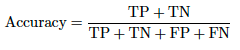Unlike Accuracy, which looks at the number of true positive and true negative predictions per class, Balanced Accuracy looks at the true positives (TP) and the false negatives (FN) for each class, also known as Recall. It is the sum of the recall values of each class divided by the total number of classes. (This formula matches the TPR formula.)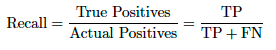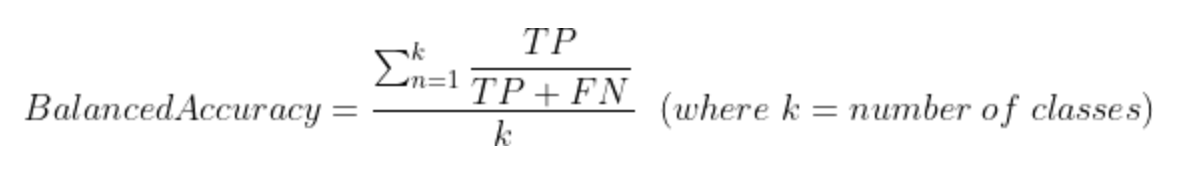For example, in the 3x3 matrix example below: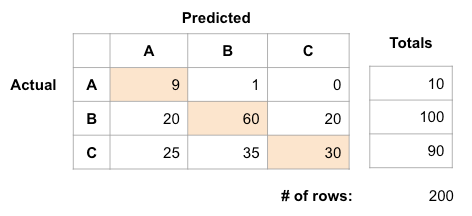Accuracy = `(TP_A + TP_B + TP_C) / Total prediction count` or, from the image above, `(9 + 60 + 30) / 200 = 0.495`

Balanced Accuracy = `(Recall_A + Recall_B + Recall_C) / total number of classes`.

``````Recall_A = 9 / (9 + 1 + 0) = 0.9
Recall_B = 60 / (20 + 60 + 20) = 0.6
Recall_C = 30 / (25 + 35 + 30) = 0.333
``````

Balanced Accuracy = `(0.9 + 0.6 +0.333) / 3 = 0.611`

Accuracy and Balanced Accuracy apply to both binary and multiclass classification.

Using weights: Every cell of the confusion matrix will be the sum of the sample weights in that cell. If no weights are specified, the implied weight is 1, so the sum of the weights is also the count of observations.

Accuracy does not perform well with imbalanced data sets. For example, if you have 95 negative and 5 positive samples, classifying all as negative gives 0.95 accuracy score. Balanced Accuracy (bACC) overcomes this problem by normalizing true positive and true negative predictions by the number of positive and negative samples, respectively, and dividing their sum into two. This is equivalent to the following formula: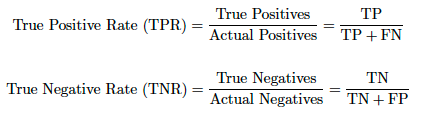### Approximate Median Significance (deprecated)¶

Display Full name Description Project type
AMS@15%tsh Approximate Median Significance Measures the median of estimated significance with a 15% threshold. Binary classification
AMS@opt_tsh Approximate Median Significance Measures the median of estimated significance with an optimal threshold. Binary classification

The Approximate Median Significance (AMS) metric creates a distinction between the two classes in a binary classification problem as the “signal” (true positive) and the remaining as the “background” (false positive). This metric was largely brought to light from the ATLAS experiment to identify the Higgs boson, and the associated Kaggle competition.

Since the probability of a signal event is usually several orders of magnitude lower than the probability of a background event, the signal and background samples are usually renormalized to produce a balanced classification problem. Next, a real-value discriminant function is trained on this reweighted sample to minimize any weighted classification error. The signal region is then defined by cutting the discriminant value at a certain threshold, which may be optimized on a held-out set to maximize the sensitivity of the statistical test.

Given a classifier, `g`, and n observed events selected by `g` (positives), the (Gaussian) significance of discovery would be, roughly:standard deviations where:is the expected value of background observations, and thus:is the expected value of signal observations.

Or, stated equivalently:would suggest an objective function of:for training `g`. However, it is only valid when `s << b and b >> 1`, which is often not the case in practice. To improve the behavior of the objective function in this range, the AMS objective function is defined by:Where `s` (signal), `b` (background): unnormalized true positive and false positive rates, respectively, is a regularization term, set as a constant equal to 10.

The classifier is trained on simulated background and signal events. The AMS `s` and `b`are the sum of signal and background weights, respectively, in the selection region, and the objective is a function of the weights of selected events. Simulators produce weights for each event to correct for the mismatch between the natural (prior) probability of the event and the instrumental probability applied by the simulator. After re-normalizing the samples to produce a balanced classification problem, a real-valued discriminant function is then trained on this reweighted sample to minimize the weighted classification error. The signal region is then defined by cutting the discriminant value at a certain threshold, which is optimized on a held-out set to maximize the sensitivity of the statistical test.

### AUC/Weighted AUC¶

Display Full name Description Project type
AUC/Weighted AUC Area Under the (ROC) Curve Measures the ability to distinguish the ones from the zeros; for multiclass, AUC is calculated for each class one-vs-all and then averaged, weighted by the class frequency. Binary classification, multiclass, multilabel

AUC for the ROC curve is a performance measurement for classification problems. ROC is a probability curve and AUC represents the degree or measure of separability. The metric ranges from 0 to 1 and indicates how much the model is capable of distinguishing between classes. The higher the AUC, the better the model is at predicting negatives (0s as 0s) and positives (1s as 1s). The ROC curve shows how the true positive rate (sensitivity) on the Y-axis and false positive rate (specificity) on the X-axis vary at each possible threshold.

For a multiclass or multilabel model, you can plot n number of AUC/ROC Curves for n number classes using One vs All methodology. For example, if there are three classes named `X`, `Y` and `Z`, there will be one ROC for `X` classified against `Y` and `Z`, another ROC for `Y` classified against `X` and `Z`, and a third for `Z` classified against `Y` and `X`. To extend the ROC curve and the Area Under the Curve to multiclass or multilabel classification, it is necessary to binarize the output.

For multiclass projects, the AUC score is the averaged AUC score for each single class (macro average), weighted by support (the number of true instances for each class). The Weighted AUC score is the averaged, sample-weighted AUC score for each single class (macro average), weighted according to the sample weights for each class `sum(sample_weights_for_class)/sum(sample_weights)`.

For multilabel projects, the AUC score is the averaged AUC score for each single class (macro average). The Weighted AUC score is the averaged, sample-weighted AUC score for each single class (macro average).

### Area Under PR Curve¶

Display Full name Description Project type
Area Under PR Curve Area Under the Precision-Recall Curve Approximation of the Area under the Precision-Recall Curve; summarizes precision and recall in one score. Well-suited to imbalanced targets. Binary classification, multilabel

The Precision-Recall (PR) curve captures the tradeoff between a model's precision and recall at different probability thresholds. Precision is the proportion of positively labeled cases that are true positives (i.e., `TP / (TP + FP)` ), and recall is the proportion of positive labeled cases that are recovered by the model (`TP/ (TP + FN)`).

The area under the PR curve cannot always be calculated exactly, so an approximation is used by means of a weighted mean of precisions at each threshold, weighted by the improvement in recall from the previous threshold: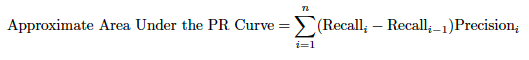Area under the PR curve is very well-suited to problems with imbalanced classes where the minority class is the "positive" class of interest (it is important that this is encoded as such): precision and recall both summarize information about positive class retrieval, and neither is informed by True Negatives.

For more reading about the relative merits of using the above approach as opposed to an interpolation of the area, see:

For multilabel projects, the reported Area Under PR Curve score is the averaged Area Under PR Curve score for each single class (macro average).

### Deviance metrics¶

Display Full name Description Project type
Gamma Deviance/ Weighted Gamma Deviance Gamma Deviance Measures the inaccuracy of predicted mean values when the target is skewed and gamma distributed. Regression
Poisson Deviance/Weighted Poisson Deviance Poisson Deviance Measures the inaccuracy of predicted mean values for count data. Regression
Tweedie Deviance/Weighted Tweedie Deviance Tweedie Deviance Measures the inaccuracy of predicted mean values when the target is zero-inflated and skewed. Regression

Deviance is a measure of the goodness of model fit—how well your model fits the data. Technically, it is how well your fitted prediction model compares to a perfect (saturated) model from the observed values. This is usually defined as twice the log-likelihood function, parameters that are determined via a maximum likelihood estimation. Thus, the deviance is defined as the difference of likelihoods between the fitted model and the saturated model. As a consequence, the deviance is always larger than or equal to zero, where zero only applies if the fit is perfect.

Deviance metrics are based on the principle of generalized linear models. That is, the deviance is some measure of the error difference between the target value and the predicted value, where the predicted value is run through a link function, denoted with: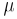An example of a link function is the logit function, which is used in logistic regression to transform the prediction from a linear model into a probability between 0 and 1. In essence, each deviance equation is an error metric intended to work with a type of distribution deemed applicable for the target data.

For example, a normal distribution for a target uses the sum of squared errors: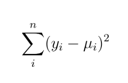And the Python implementation: `np.sum((y - pred) ** 2)`

In this case, the deviance metric is just that—the sum of squared errors.

For a Gamma distribution, where data is skewed to one side (say to the right for something like the distribution of how much customers spend at a store), deviance is: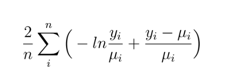Python: `2 * np.mean(-np.log(y / pred) + (y - pred) / pred)`

For a Poisson distribution, when interested in predicting counts or number of occurrences of something, the function is this: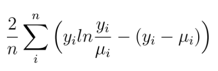Python: `2 * np.mean(y * np.log(y / pred) - (y - pred))`

For Tweedie, the function looks a little messier. Tweedie Deviance measures how well the model fits the data, assuming the target has a Tweedie distribution. Tweedie is commonly used in zero-inflated regression problems, where there are a relatively large number of 0s and the rest are continuous values. Smaller values of Deviance are more accurate models. As Tweedie Deviance is a more complicated metric, it may be easier to explain the model using FVE (Fraction of Variance Explained) Tweedie. This metric is equivalent to R^2, but for Tweedie distributions instead of Normal distributions. A score of 1 is a perfect explanation.

Tweedie deviance attempts to differentiate between a variety of distribution families, including Normal, Poisson, Gamma, and some less familiar distributions. This includes a class of mixed compound Poisson–Gamma distributions that have positive mass at zero, but are otherwise continuous (e.g., zero-inflated distributions). In this case, the function is: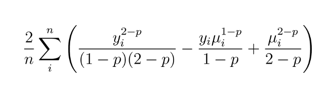Python: `2 * np.mean((y ** (2-p)) / ((1-p) * (2-p)) - (y * (pred ** (1-p))) / (1-p) + (pred ** (2-p)) / (2-p))`

Where parameter `p` is an index value that differentiates between the distribution family. For example, 0 is Normal, 1 is Poisson, 1.5 is Tweedie, and 2 is Gamma.

Interpreting these metric scores is not particularly intuitive. `y` and `pred` values are in the unit of target (e.g., dollars), but as can be seen above, log functions and scaling complicates it.

You can transform this to a weighted deviance function simply by introducing a weights multiplier, for example for Poisson: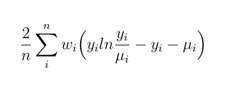Note

Because of log functions and predictions in the denominator in some calculations, this only works for positive responses. That is, predictions are enforced to be strictly positive `(max(pred, 1e-8))` and actuals are enforced to be either non-negative `(max(y, 0))` or strictly positive `(max(y, 1e-8))`, depending on the deviance function.

### FVE deviance metrics¶

Display Full name Description Project type
FVE Binomial/Weighted FVE Binomial Fraction of Variance Explained Measures deviance based on fitting on a binomial distribution. Binary classification
FVE Gamma/Weighted FVE Gamma Fraction of Variance Explained Measures FVE for gamma deviance. Regression
FVE Multinomial/Weighted FVE Multinomial Fraction of Variance Explained Measures deviance based on fitting on a multinomial distribution. Multiclass
FVE Poisson/Weighted FVE Poisson Fraction of Variance Explained Measures FVE for Poisson deviance. Regression
FVE Tweedie/Weighted FVE Tweedie Fraction of Variance Explained Measures FVE for Tweedie deviance. Regression

FVE is fraction of variance explained (also sometimes referred to as "fraction of deviance explained"). That is, what proportion of the total deviance, or error, is captured by the model? This is defined as:

To calculate the fraction of variance explained, three models are fit:

• The "model analyzed," or the model actually constructed within DataRobot.
• A "worst fit" model (a model fitted without any predictors, fitting only an intercept).
• A "perfect fit" model (also called a "fully saturated" model), which exactly predicts every observation.

"Null deviance" is the total deviance calculated between the "worst fit" model and the "perfect fit" model. "Residual deviance" is the total deviance calculated between the "model analyzed" and the "perfect fit" model. (See the deviance formulas for more detail.)

You can think of the "fraction of unexplained deviance" as the residual deviance (a measure of error between the "perfect fit" model and your model) divided by the null deviance (a measure of error between the "perfect fit" model and the "worst fit" model). The fraction of explained deviance is 1 minus the fraction of unexplained deviance. Gauge the model's performance improvement compared to the "worst fit" model by calculating an R²-style statistic, the Fraction of Variance Explained (FVE).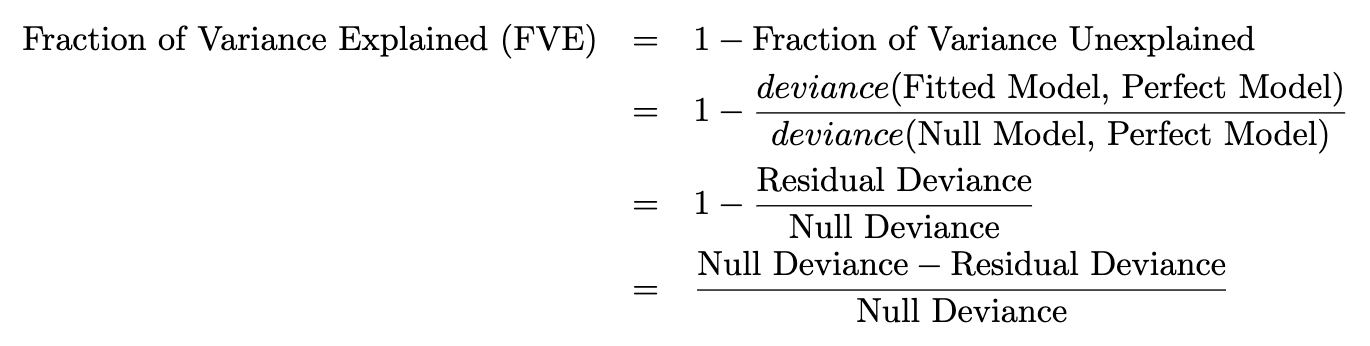Illustrated conceptually as: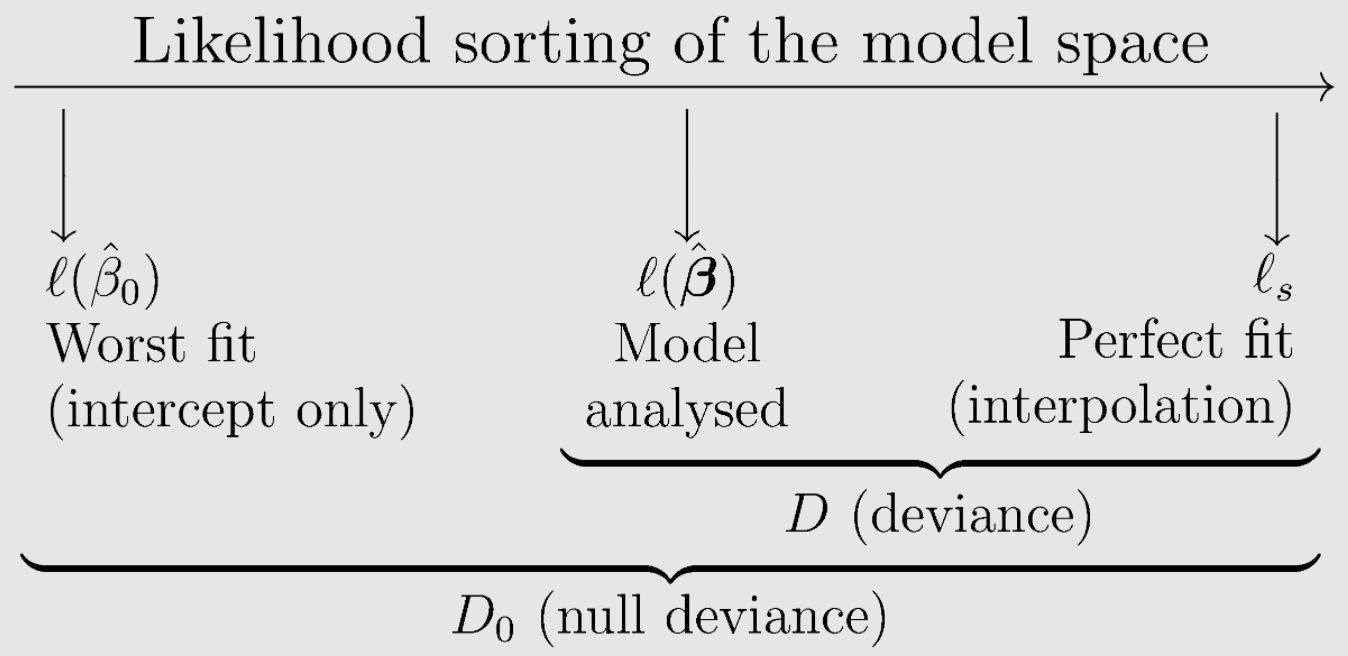* Illustration courtesy of Eduardo García-Portugués, Notes for Predictive Modeling.

Therefore, FVE equals traditional R-squared for linear regression models, but, unlike traditional R-squared, generalizes to exponential family regression models. By scaling the difference by the Null Deviance, the value of FVE should be between 0 and 1, but not always. It can be less than zero in the event the model predicts responses poorly for new observations and/or a cross-validated out of sample data is very different.

For multiclass projects, FVE Multinomial computes `loss = logloss(act, pred)` and `loss_avg = logloss(act, act_avg)`, where:

• `act_avg` is the one-hot encoded "actual" data.
• each class (column) is averaged over `N` data points.

Basically `act_avg` is a list containing the percentage of the data that belongs to each class. Then, the FVE is computed via `1 - loss / loss_avg`.

### Gini coefficient¶

Display Full name Description Project type
Gini/Weighted Gini Gini Coefficient Measures the ability to rank. Regression, binary classification
Gini Norm/Weighted Gini Norm Normalized Gini Coefficient Measures the ability to rank. Regression, binary classification

In machine learning, the Gini Coefficient or Gini Index measures the ability of a model to accurately rank predictions. Gini is effectively the same as AUC, but on a scale of -1 to 1 (where 0 is the score of a random classifier). If the Gini Norm is 1, then the model perfectly ranks the inputs. Gini can be useful when you care more about ranking your predictions, rather than the predicted value itself.

Gini is defined as a ratio of normalized values between 0 and 1—the numerator as the area between the Lorenz curve of the distribution and the 45 degree uniform distribution line, discussed below.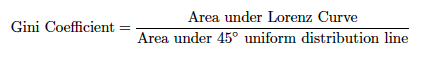The Gini coefficient is thus defined as the blue area divided by the area of the lower triangle: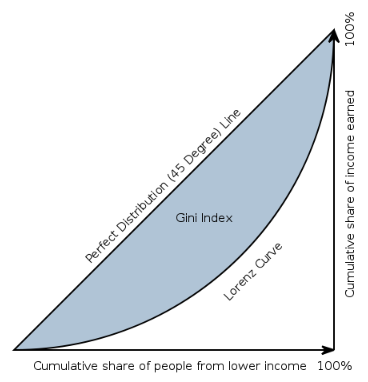The Gini coefficient is equal to the area below the line of perfect equality (0.5 by definition) minus the area below the Lorenz curve, divided by the area below the line of perfect equality. In other words, it is double the area between the Lorenz curve and the line of perfect equality. The line at 45 degrees thus represents perfect equality. The Gini coefficient can then be thought of as the ratio of the area that lies between the line of equality and the Lorenz curve (call that `A`) over the total area under the line of equality (call that `A + B`). So:

`Gini = A / (A + B)`

It is also equal to `2A` and to `1 − 2B` due to the fact that `A + B = 0.5` (since the axes scale from 0 to 1).

It is alternatively defined as twice the area between the receiver operating characteristic (ROC) curve and its diagonal, in which case the AUC (Area Under the ROC Curve) measure of performance is given by `AUC = (G + 1)/2` or factored as `2 * AUC-1`.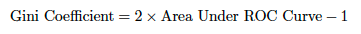Its purpose is to normalize the AUC so that a random classifier scores 0, and a perfect classifier scores 1. Formally then, the range of possible Gini coefficient scores is [-1, 1] but in practice zero is typically the low end. You can also integrate the area between the perfect 45 degree line and the Lorenz curve to get the same Gini value, but the former is arguably easier.

In economics, the Gini coefficient is a measure of statistical dispersion intended to represent the income or wealth distribution of a nation's residents and is the most commonly used measure of inequality. A Gini coefficient of zero expresses perfect equality, where all values are the same (for example, where everyone has the same income). In this context, a Gini coefficient of 1 (or 100%) expresses maximal inequality among values (e.g., for a large number of people, where only one person has all the income or consumption, and all others have none, the Gini coefficient will be very nearly one). However, a value greater than 1 can occur if some persons represent negative contribution to the total (for example, having negative income or wealth). Using this economics example, the Lorenz curve shows income distribution by plotting the population percentile by income on the horizontal axis and cumulative income on the vertical axis. The Normalized Gini Coefficient adjusts the score by the theoretical maximum so that the maximum score is 1. Because the score is normalized, comparisons can be made between the Gini coefficient values of like entities such that values can be rank ordered. For example, economic inequality by country is commonly assessed with the Gini coefficient and is used to rank order the countries:

Rank Country Distribution of family income—Gini index Date of information
1 LESOTHO 63.2 1995
2 SOUTH AFRICA 62.5 2013 EST.
3 MICRONESIA, FEDERATED STATES OF 61.1 2013 EST.
4 HAITI 60.8 2012
5 BOTSWANA 60.5 2009

One way to use the Gini index metric in a machine learning context is to compute it using the actual and predicted values, instead of using individual samples. If, using the example above, you generate the Gini index from the samples of individual incomes of people in a country, the Lorenz curve is a function of the population percentage by cumulative sum of incomes. In a machine learning context, you could generate the Gini from the actual and predicted values. One approach would be to pair the actual and predicted values and sort them by predicted. The Lorenz curve in that case is a function of the predicted values by the cumulative sum of actuals—the running total of the 1s of class 1 values. Then, calculate the Gini using one of the formulas above.

For an example, see the Porto Seguro’s Safe Driver Kaggle competition and the corresponding explanation.

### Kolmogorov–Smirnov (KS)¶

Display Full name Description Project type
KS Kolmogorov-Smirnov Measures the maximum distance between two non-parametric distributions. Used for ranking a binary classifier, KS evaluates models based on the degree of separation between true positive and false positive distributions. The KS value is displayed in the ROC Curve. Binary classification

The KS or Kolmogorov-Smirnov chart measures performance of classification models. More accurately, KS is a measure of the degree of separation between positive and negative distributions. The KS is 1 if the scores partition the population into two separate groups in which one group contains all the positives and the other all the negatives. On the other hand, If the model can’t differentiate between positives and negatives, then it is as if the model selects cases randomly from the population. In that case, the KS would be 0. In most classification models, the KS will fall between 0 and 1; the higher the value, the better the model is at separating the positive from negative cases.

In this paper, in binary classification problems, it has been used as dissimilarity metric for assessing the classifier’s discriminant power measuring the distance that its score produces between the cumulative distribution functions (CDFs) of the two data classes, known as KS2 for this purpose (two samples). The usual metric for both purposes is the maximum vertical difference (MVD) between the CDFs (the Max_KS), which is invariant to score range and scale making it suitable for classifiers comparisons. The MVD is simply the vertical distance between the two curves at a single point on the X axis. The Max_KS is the single point where this distance is the greatest.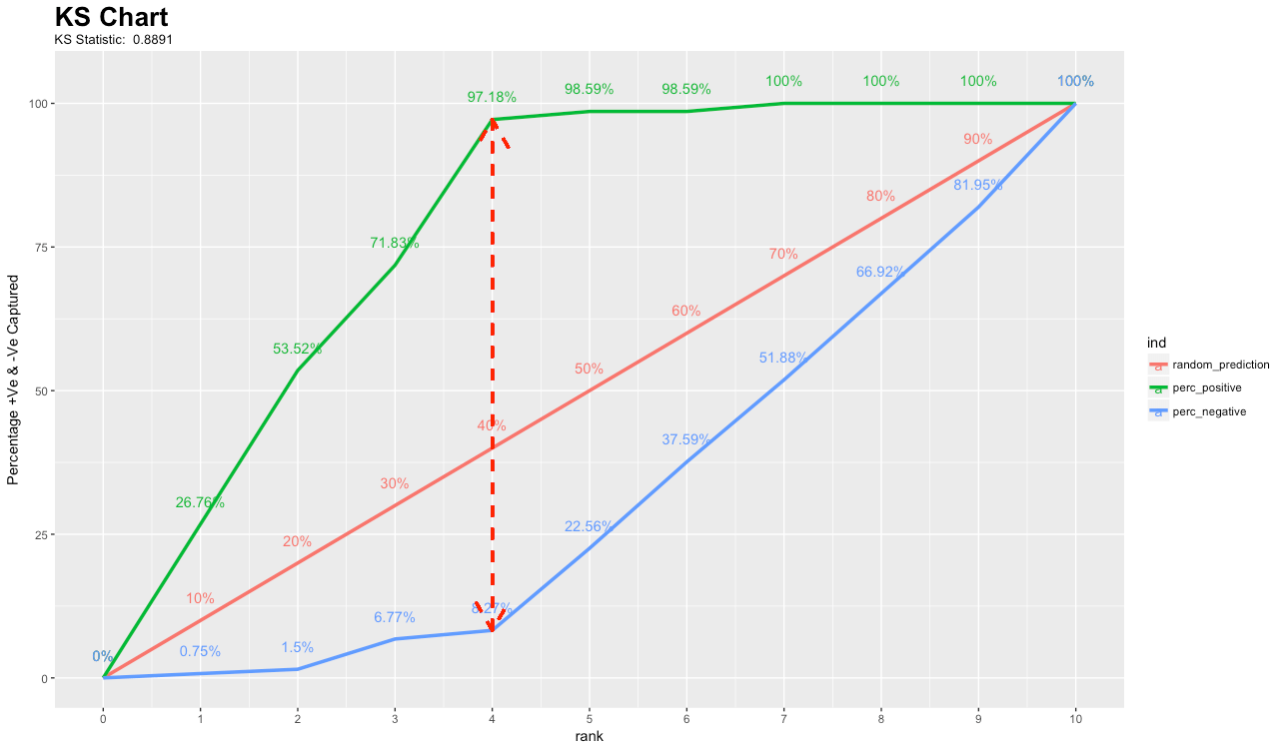### LogLoss/Weighted LogLoss¶

Display Full name Description Project type
LogLoss/ Weighted LogLoss Logarithmic Loss Measures the inaccuracy of predicted probabilities. Binary classification, multiclass, multilabel

Cross-entropy loss, or log loss, measures the performance of a classification model whose output is a probability value between 0 and 1. Log loss increases as the predicted probability diverges from the actual label. So, for example, predicting a probability of .12 when the actual observation label is 1, or predicting .91 when the actual observation label is 0, would be “bad” and result in a higher loss value than misclassification probabilities closer to the true label value. A perfect model would have a log loss of 0.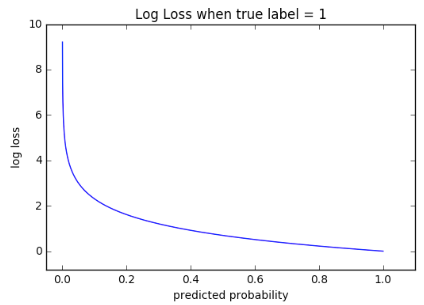The graph above shows the range of possible loss values given a true observation (true = 1). As the predicted probability approaches 1, log loss slowly decreases. As the predicted probability decreases, however, the log loss increases rapidly. Log loss penalizes both types of errors, but especially those predictions that are confident and wrong.

Cross-entropy and log loss are slightly different depending on context, but in machine learning when calculating error rates between 0 and 1 they resolve to the same thing.

In binary classification, the formula equals `-(ylog(p) + (1 - y)log(1 - p))` or: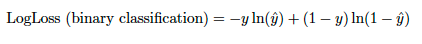Where `p` is the predicted value of `y`.

Similarly for multiclass and multilabel, take the sum of log loss values for each class prediction in the observation: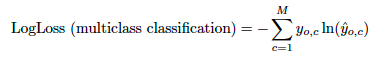You can transform this to a weighted loss function by introducing weights to a given class: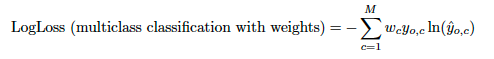Note that the reported log loss scores for multilabel are scaled by `1/number_of_unique_classes`.

### MAE/Weighted MAE¶

Display Full name Description Project type
MAE/Weighted MAE Mean Absolute Error Measures the inaccuracy of predicted median values. Regression

DataRobot implements a MAE metric using the median to measure absolute deviations, which is a more accurate calculation of absolute deviance (or rather stated, absolute error in this case). This is based on the fact that in optimizing the loss function for the absolute error, the best value that is derived turns out to be the median of the series.

To see why, first assume a series of numbers that you want to summarize to an optimal value, (`x1,x2,…,xn`)— the predictions. You want the summary to be a single number, `s`. How do you select `s` so that it summarizes the predictions, (`x1,x2,…,xn`), effectively? Aggregate the error deviances between `xi`and `s` for each of `xi` into a single summary of the quality of a proposed value of `s`. To perform this aggregation, sum up the deviances over each of the `xi` and call the result `E`: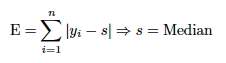Upon solving for the `s` that results in the smallest error, the `E` loss function optimizes to be the median, not the mean. Note that, likewise, the best value of the squared error loss function optimizes to be the mean. Thus, the mean squared error.

While MAE stands for “mean absolute error,” it optimizes the model to predict the median correctly. This is similar to how RMSE is “root mean squared error,” but optimizes for predicting the mean correctly (not the square of the mean).

You may notice some curious discrepancies in DataRobot, which are worth remembering, when you optimize for MAE. Most insights report for the mean. As such, all the Lift Charts look “off” because the model under- or over-predicts for every point along the distribution. The Lift Chart calculates a mean, whereas MAE optimizes for the median.

You can transform this to a weighted loss function by introducing weights to observations: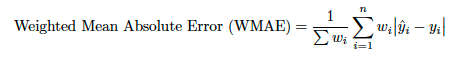Unfortunately, the statistical literature has not yet adopted a standard notation, as both the mean absolute deviation around the mean (MAD) and the mean absolute error (what DataRobot calls “MAE”) have been denoted by their initials MAD in the literature, which may lead to confusion, since in general, they can have values considerably different from each other.

### MAPE/Weighted MAPE¶

Display Full name Description Project type
MAPE/Weighted MAPE Mean Absolute Percentage Error Measures the percent inaccuracy of the mean values. Regression

One problem with the MAE is that the relative size of the error is not always obvious. Sometimes it is hard to tell a large error from a small error. To deal with this problem, find the mean absolute error in percentage terms. Mean Absolute Percentage Error (MAPE) allows you to compare forecasts of different series in different scales. For example, consider comparing the sales forecast accuracy of one store with the sales forecast of another, similar store, even though these stores may have different sales volumes.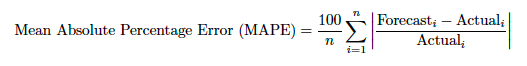### MASE¶

Display Full name Description Project type
MASE Mean Absolute Scaled Error Measures relative performance with respect to a baseline model. Regression (time series only)

MASE is a measure of the accuracy of forecasts and is a comparison of one model to a naïve baseline model—the simple ratio of the MAE of a model over the baseline model. This has the advantage of being easily interpretable and explainable in terms of relative accuracy gain, and is recommended when comparing models. In DataRobot time series projects, the baseline model is a model that uses the most recent value that matches the longest periodicity. That is, while a project could have multiple different naïve predictions with different periodicity, DataRobot uses the longest naïve predictions to compute the MASE score.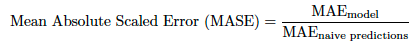Or in more detail: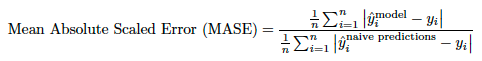Where the numerator is the model of interest and the denominator is the naïve baseline model.

### Max MCC/Weighted Max MCC¶

Display Full name Description Project type
Max MCC/Weighted Max MCC Maximum Matthews correlation coefficient Measures the maximum value of the Matthews correlation coefficient between the predicted and actual class labels. Binary classification

Matthews correlation coefficient is a balanced metric for binary classification that takes into account all four entries in the confusion matrix. It can be calculated as: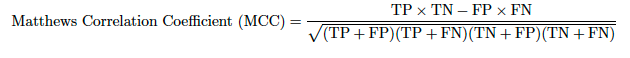Where:

Outcome Description
True positive (TP) A positive instance that the model correctly classifies as positive.
False positive (FP) A negative instance that the model incorrectly classifies as positive.
True negative (TN) A negative instance that the model correctly classifies as negative.
False negative (FN) A positive instance that the model incorrectly classifies as negative.

The range of possible values is [-1, 1], where 1 represents perfect predictions.

Since the entries in the confusion matrix depend on the prediction threshold, DataRobot uses the maximum value of MCC over possible prediction thresholds.

### R-Squared (R2)/Weighted R-Squared¶

Display Full name Description Project type
R-Squared/Weighted R-Squared R-Squared Measures the proportion of total variation of outcomes explained by the model. Regression

R-squared is a statistical measure of goodness of fit—how close the data are to a fitted regression line. It is also known as the coefficient of determination, or the coefficient of multiple determination for multiple regression. As a description of the variance explained, it is the percentage of the response variable variation that is explained by a linear model. Typically R-squared is between 0 and 100%. 0% indicates that the model explains none of the variability of the response data around its mean. 100% indicates that the model explains all the variability of the response data around its mean.

Note that there are circumstances that result in a negative R value, meaning that the model is predicting worse than the mean. This can happen, for example, due to problematic training data. For time-aware projects, R-squared has a higher chance to be negative due to mean changes over time—if you train a model on a high mean period, but test on a low mean period, a large negative R-squared value can result. (When partitioning is done via random sampling, the target mean for the train and test sets are roughly the same, so negative R-squared values are less likely.) Generally speaking, it is best to avoid models with a negative R-squared value.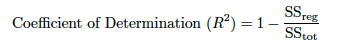Where `SS_res` is the residual sum of squares, also called the explained sum of squares: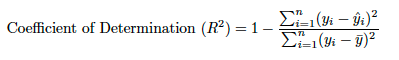`SS_tot` is the total sum of squares (proportional to the variance of the data) and: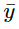is the sample mean of `y`, calculated from the training data: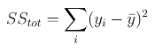For a weighted R-squared, `SS_res` becomes: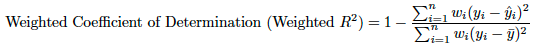And `SS_tot` becomes: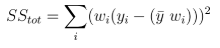Some key Limitations of R-squared:

• R-squared cannot determine whether the coefficient estimates and predictions are biased, which is why you must assess the residual plots.

• R-squared can be artificially made high. That is, you can increase the value of R-squared by simply adding more and more independent variables to the model. In other words, R-squared never decreases upon adding more independent variables. Sometimes, some of these variables might be very insignificant and can be really useless to the model.

• R-squared does not indicate whether a regression model is adequate. You can have a low R-squared value for a good model, or a high R-squared value for a model that does not fit the data. To that end, R-squared values must be interpreted with caution.

Low R-squared values aren’t inherently bad. In some fields, it is entirely expected that your R-squared values will be low. For example, any field that attempts to predict human behavior, such as psychology, typically has R-squared values lower than 50%. Humans are simply harder to predict than, say, physical processes.

At the same time, high R-squared values aren’t inherently good. A high R-squared does not necessarily indicate that the model has a good fit. For example, the fitted line plot may indicate a good fit and seemingly express the high R-squared, but a look at the residual plot may show a systematic over and/or under prediction, indicative of high bias.

DataRobot calculates on out-of-sample data, mitigating traditional critiques such as, for example, that adding more features increases the value or that R2 is not applicable to non-linear techniques. It is essentially treated as a scaled version of RMSE, allowing DataRobot to compare itself to the mean model (R2 = 0) and determine if it’s doing better (R2 >0) or worse (R2 <0).

### Rate@Top10%, Rate@Top5%, Rate@TopTenth%¶

Display Full name Description Project type
Rate@Top5% Rate@Top5% Measures the response rate in the top 5% highest predictions. Binary classification
Rate@Top10% Rate@Top10% Measures the response rate in the top 10% highest predictions. Binary classification
Rate@TopTenth% Rate@TopTenth% Measures the response rate in the top 0.1% highest predictions. Binary classification

Rate@Top5%, Rate@Top10%, and Rate@TopTenth% are a measure of accuracy for a classification model and are simply the calculations of the accuracy for the top 5%, top 10% and top tenth% of highest predictions, respectively. For example, take a set of 100 predictions ordered from lowest to highest, something like: [.05, .08, .11, .12, .14 … .87, .89, .91, .93, .94 ]. Presuming the threshold is below .87, the top 5 predictions from .87 to .94 would be assigned to the positive class, 1. Now say the actual values for the top 5 are [1, 1, 0, 1, 1]. Then the Rate@Top5% measure of accuracy would be 80%.

### RMSE, Weighted RMSE & RMSLE, Weighted RMSLE¶

Display Full name Description Project type
RMSE/ Weighted RMSE Root Mean Squared Error Measures the inaccuracy of predicted mean values when the target is normally distributed. Regression, binary classification
RMSLE/ Weighted RMSLE* Root Mean Squared Log Error Measures the inaccuracy of predicted mean values when the target is skewed and log-normal distributed. Regression

The root mean squared error (RMSE) is another measure of accuracy somewhat similar to MAD in that they both take the difference between the actual and the predicted or forecast values. However, RMSE squares the difference rather than applying the absolute value, and then finds the square root.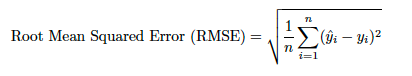Thus, RMSE is always non-negative and a value of 0 indicates a perfect fit to the data. In general, a lower RMSE is better than a higher one. However, comparisons across different types of data would be invalid because the measure is dependent on the scale of the numbers used.

RMSE is the square root of the average of squared errors. The effect of each error on RMSE is proportional to the size of the squared error. Thus, larger errors have a disproportionately large effect on RMSE. Consequently, RMSE is sensitive to outliers.

The root mean squared log error (RMSLE), to avoid taking the natural log of zero, adds 1 to both actual and predicted before taking the natural logarithm. As a result, the function can be used if actual or predicted have zero-valued elements. Note that only the percent difference between the actual and prediction matter. For example, P = 1000 and A = 500 would give roughly the same error as when P = 100000 and A = 50000.You can transform this to a weighted function simply by introducing a weights multiplier: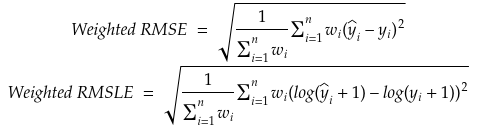Note

For RMSLE, many model blueprints log transform the target and optimize for RMSE. This is equivalent to optimizing for RMSLE. If this occurs, the model's build information lists "log transformed response".

### Silhouette Score¶

Display Full name Description Project type
Silhouette Score Silhouette score, also referred to as silhouette coefficient Compares clustering models. Clustering

The silhouette score, also called the silhouette coefficient, is a metric used to compare clustering models. It is calculated using the mean intra-cluster distance (average distance between each point within a cluster) and the mean nearest-cluster distance (average distance between clusters). That is, it takes into account the distances between the clusters, but also the distribution of each cluster. If a cluster is condensed, the instances (points) have a high degree of similarity. The silhouette score ranges from -1 to +1. The closer to +1, the more separated the clusters are.

Computing silhouette score for large datasets is very time-intensive—training a clustering model takes minutes but the metric computation can take hours. To address this, DataRobot performs stratified sampling to limit the dataset to 50000 rows so that models are trained and evaluated for large datasets in a reasonable timeframe while also providing a good estimation of the actual silhouette score.

In time series, the silhouette score is a measure of the silhouette coefficient between different series calculated by comparing the similarity of the data points across the different series. Similar to non-time series use cases, the distance is calculated using the distances between the series; however, there is an important distinction in that the silhouette coefficient calculations do not account for location in time when considering similarity.

While the silhouette score is generally useful, consider it with caution for time series. The silhouette score can identify series that have a high degree of similarity in the points contained within the series, but it does not account for periodicity and trends, or similarities across time.

To understand the impact, examine the following two scenarios:

#### Silhouette time series scenario 1¶

Consider these two series:

• The first series has a large spike in the first 10 points, followed by 90 small to near-zero values.

• The second series has 70 small to near-zero values followed by a moderate spike and several more near-zero values.

In this scenario, the silhouette coefficient will likely be large between these two series. Given that time isn't taken into account, the values show a high degree of mathematical similarity.

#### Silhouette time series scenario 2¶

Consider these three series:

• The first series is a sine wave of magnitude 1.

• The second series is a cosine wave of magnitude 1.

• The third series is a cosine wave of magnitude 0.5.

Potential clustering methods:

• The first method adds the sine and cosine wave (both having a magnitude of 1) into a cluster and it adds the smaller cosine wave into a second cluster.

• The second method adds the two cosine waves into a single cluster and the sine wave into a separate cluster.

The first method will likely have a higher silhouette score than the second method. This is because the silhouette score does not consider the periodicity of the data and the fact that the peaks in the cosine waves likely have more meaning to each other.

If the goal is to perform segmented modeling, take the silhouette score into consideration, but be aware of the following:

• A higher silhouette score may not indicate a better segmented modeling performance.
• Series grouped together based on periodicity, volatility, or other time-dependent features will likely return lower silhouette scores than series that have a higher similarity when considering only the magnitudes of values independent of time.

### SMAPE/Weighted SMAPE¶

Display Full name Description Project type
SMAPE/Weighted SMAPE Symmetric Mean Absolute Percentage Error Measures the bounded percent inaccuracy of the mean values. Regression

The Mean Absolute Percentage Error (MAPE) allows you to compare forecasts of different series in different scales. However, MAPE cannot be used if there are zero values, and does not have an upper limit to the percentage error. In these cases, the Symmetric Mean Absolute Percentage Error (SMAPE) can be a good alternative. The SMAPE has a lower and upper boundary, and will always result in a value between 0% and 200%, which makes statistical comparisons between values easier. It is also a suitable function for use on data where values that are zero are present. That is, rows in which `Actual = Forecast = 0`, DataRobot replaces `0/0 = NaN` with zero before summing over all rows.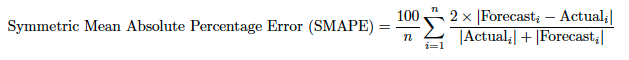### Theil's U¶

Display Full name Description Project type
Theil's U Henri Theil's U Index of Inequality Measures relative performance with respect to a baseline model. Regression (time series only)

Theil’s U, similar to MASE, is a metric to evaluate the accuracy of a forecast relative to the forecast of the naïve model (a model that uses, for predictions, the most recent value that matches the longest periodicity).

This has the advantage of being easily interpretable and explainable in terms of relative accuracy gain, and is recommended when comparing models. In DataRobot time series projects, the baseline model is a model that uses the most recent value that matches the longest periodicity. That is, while a project could have multiple different naïve predictions with different periodicity, DataRobot uses the longest naïve predictions to compute the Theil's U score.

The comparison of the forecast model to the naïve model is a function of the ratio of the two. A value greater or less than 1 indicates the model is worse or better than the naïve model, respectively.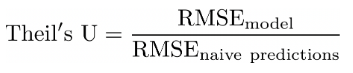Or, in more detail: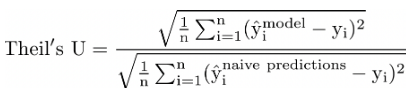Where the numerator is the model of interest and the denominator is the naïve baseline model.

Updated March 21, 2023